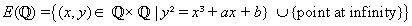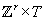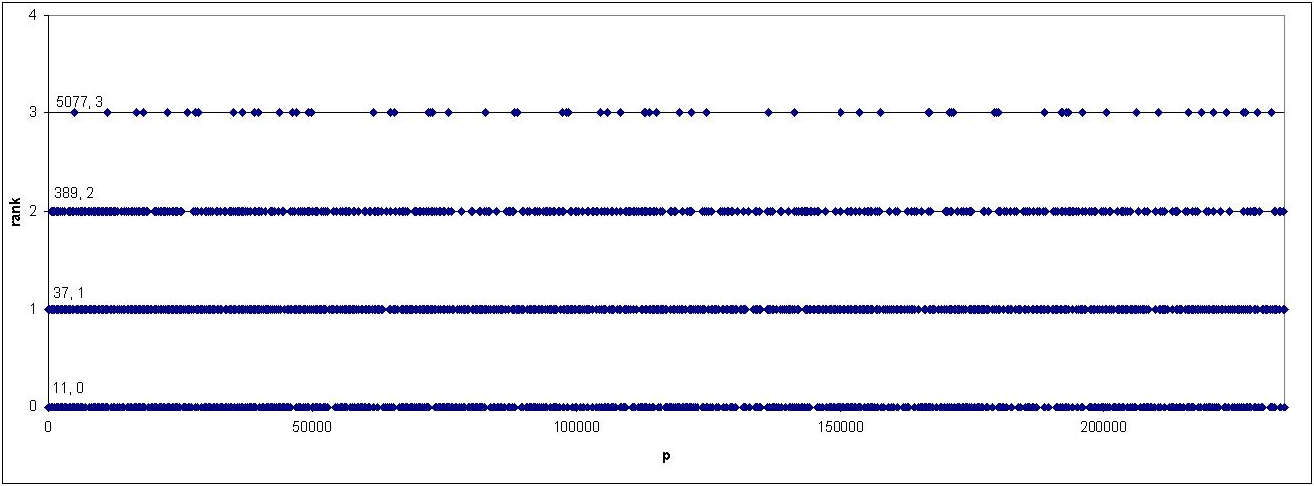# The Smallest Conductor for an Elliptic Curve of Rank Four is Composite

### Introduction

If E is an elliptic curve over Q in Weierstrass normal form y² = x³ + ax + b, then the sethas a natural structure of finitely-generated abelian group, which can be decomposed as, where T is a finite abelian group and r is greater than or equal to 0. This r is called the rank of the curve.

The conductor N of an elliptic curve is an integer attached to the elliptic curve which measures the extent to which E fails to define an elliptic curve over Fp for each prime p. The conductor has the following important properties:

• The conductor is divisible by the same primes as the minimal discriminant of E.
• There are only finitely many elliptic curves with a given conductor.
• The modularity theorem of Wiles et al., which was used in the proof of Fermat's Last Theorem, implies that there is an algorithm to compute all elliptic curves of a given conductor N. The modularity theorem asserts that if E is an elliptic curve of conductor N, then E is a quotient of a certain moduli curve called X0(N). There is an algorithm due to Manin and Birch for listing all elliptic curve quotients of X0(N). John Cremona has used this algorithm to compute all elliptic curves of conductor N up to 20000.

Thus, for any positive integer r, the following question can be answered in a finite amount of time:

Question 1. What the smallest N for which there is an elliptic curve of conductor N and rank r?

The smallest conductors for r = 0,1,2,3 are known:

 r smallest conductor an E of conductor N and rank r 0 11 y² + y = x³ - x² 1 37 y² + y = x³ - x 2 389 y² + y = x³ +x² - 2x 3 5077 y² + y = x³ - 7x + 6

The answer is not known with certainty for any r larger than 3. Tom Womack is maintaining a table of the current status of this problem. Below is a graph of the known conductors and ranks of the associated elliptic curves:The current smallest known conductors (as of August 2003) for curves of ranks 4, 5, and 6 are given in the following table:

 r smallest known conductor an E of conductor N and rank r 4 2 · 117223 y² + xy = x³ - x² - 79x + 289 5 19047851 y² + y = x³ - 79x + 342 6 2 · 3 · 2777 · 311341 y² + xy = x³ +x² - 2582x +48720

Notice that the smallest conductors for ranks r less than 4 are all prime. Up until August of 2003, the smallest known conductor of a curve of rank 4 was the composite 2 · 117223 = 234446. There are 20782 primes less than 234446, the largest of these being 234431. This motivated our investigation:

Question 2. Is there an elliptic curve of prime conductor less than or equal to 234431 wtih rank 4?

Much groundwork in this area was laid in the works of Brumer-McGuinness and Stein-Watkins. Our project concerns the implementation of an algorithm due to Mestre and Oesterle that can be used to quickly find all the elliptic curves of prime conductor p.

### Citations

algorithm due to Mestre and Oesterle: paper available